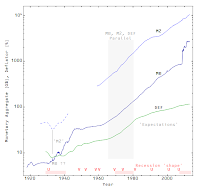## Friday, September 27, 2013

### Top ten successes of the information transfer model(1) Explaining supply and demand: If you set up a description of an information transfer process moving information through a channel from a source to a destination and make some identifications (the demand as the information source, the supply as the information destination and the price as a detector of a signal sent from the demand to the supply), you basically get out fundamental economic supply and demand logic including supply and demand diagrams (another way to visualize diagrams is here). I've used the notation Price:Demand→Supply to describe these models of a market.

(2) Modeling the price level: This is basically done with a quantity theory of money, using the information transfer framework with a market P:NGDP→MB (the price level detects signals from the aggregate demand or NGDP to the monetary base). The major results for the US are here. The fit to RGDP growth is rather remarkable. I also fit the data for the entire period from 1929 to 2013 using three monetary policy regimes with great success (market-based systems before and after WWII, with a pegged interest rate model in between). More on monetary regimes is here including a theorem that increasing the monetary base will eventually lead to an information trap (basically a liquidity trap). One of the key pieces of the model that allows such success in fitting the data is the way the behavior of money as a unit of account is incorporated in the model via a varying information transfer index. In a sense, the monetary base is the number base you count the GDP in much like binary is the number base you count data in, but in the latter case the base is fixed at two. It also works for the EU and Japan.

(3) Modeling interest rates: The best result was the same fit the data from 1960-2013 also fit 1929-2013. Overall, the simple model (derived from the IS-LM model) does a good job of describing the US, Japan and the EU.

(4) Modeling Japan's lost decade: Japan's sluggish economy and immunity to inflation is a major problem in economics. The information transfer model describes the price level and interest rates and the major conclusion is that Japan is in an information trap, a condition where monetary policy has little influence on the price level.(5) Deriving the quantity theory of money (and explaining deviations): The basic equation in the information transfer model for the market P:NGDP→MB looks like the equation of exchange. You can also derive the major result of the quantity theory from the model (specifically that for high inflation, the rate of monetary base growth equals the rate of inflation or r = i). But it is even better than that. The information transfer model explains the deviations at low inflation from the basic r = i picture.

(6) Deriving the IS-LM model: The supply and demand framework allows a straightforward derivation of the curves in an IS-LM diagram.

(7) Okun's law: A relatively straightforward application of the model building capability of the information transfer model allows us to build a market P:NGDP→LS (price level detecting a signal from the aggregate demand to the labor supply) that recovers Okun's law.

(8) Walras' law: This follows from the supply and demand model via some algebra.

(9) Explaining how the EU can be in a liquidity trap but not at the zero lower bound: Keynes' original work allowed a liquidity trap to occur at any interest rate. Later economists argued that it could only occur at zero interest rates (as they can't be lowered). Now Paul Krugman argues that the EU is in a liquidity trap even though EU rates are not actually zero (as Scott Sumner points out); Krugman's explanation is that they are close enough. The information transfer model shows that the liquidity trap rate is actually a function of GDP and the monetary base. For example, it is about 2% for the EU, 1% for Japan and 0.1% for the US.(10) Explaining the history of economic thought in the US since the early 1900s: Since the path of GDP and the monetary base give us interest rates and the price level since the early 1900s, we can use the fact that the model appears to be a quantity theory of money and an IS-LM model at different times to understand the currents in the history of economic theory. In particular, we expect to get quantity theories before the depression (check) displaced by interest rate and liquidity trap theories in the 1930s (check), a resurgence of quantity theories in the 1960s-70s (check) and a return to interest rate and liquidity trap theories today (check).

1.I should point out that in item #10 there are really three theories we are looking at ... the information transfer ISLM model, which always works but has larger empirical fluctuations (see item #3) than the second theory, the information transfer quantity theory (see item #2) which also always works. The third theory which doesn't work all the time is the traditional quantity theory (it only works for high inflation, see item #5) but as is mentioned in item #10, it works better than the ISLM model during periods of high inflation.

2.I think I read some where that you could test algorithm this way.

You apply it to one partial period of the time series and see if it predicts the nonfit period(s) of the series.

I wonder how your results done that way would work?